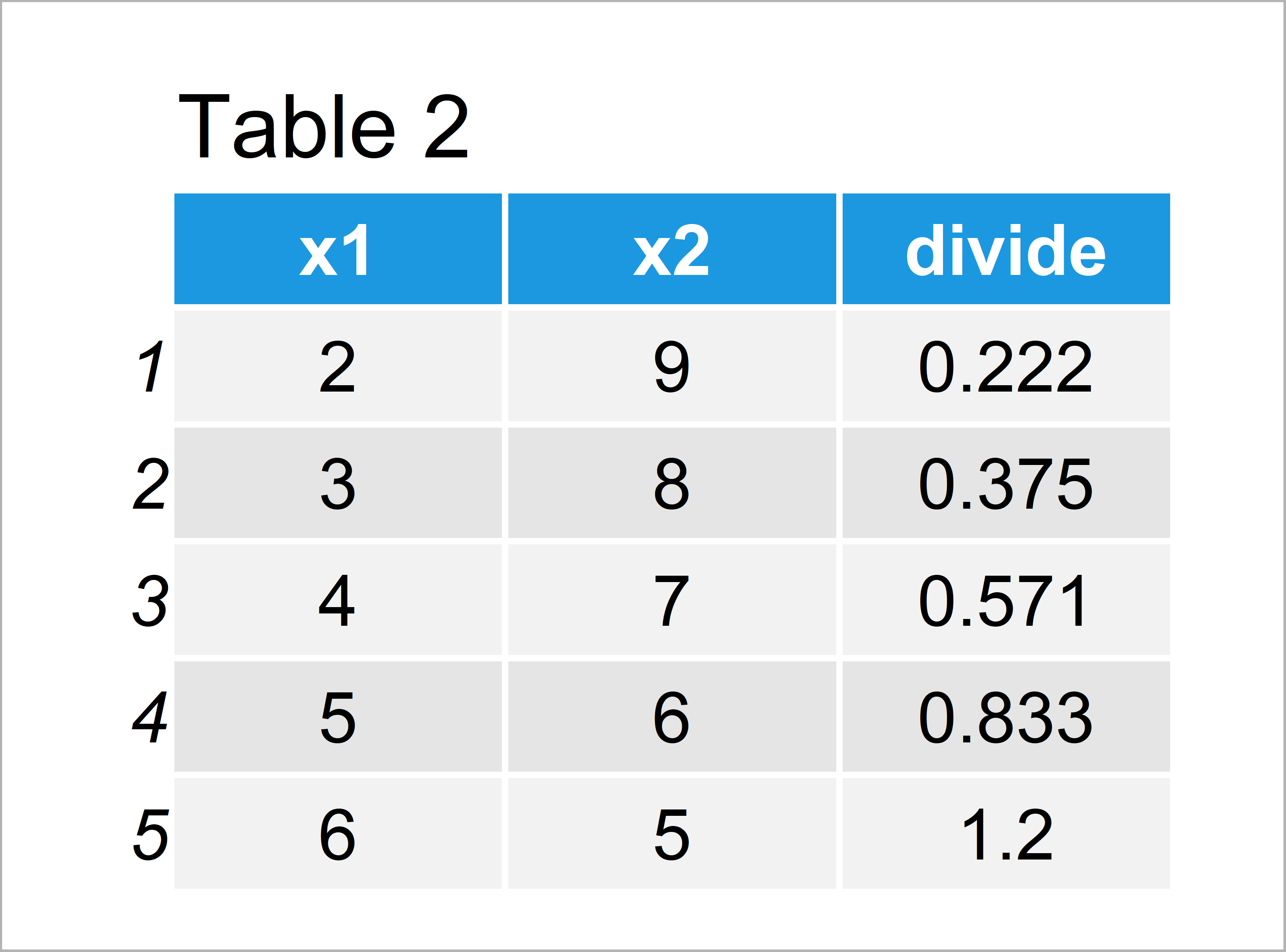# Divide One Column of Data Frame Through Another in R (2 Examples)

This tutorial shows how to divide one column of a data frame through another column of this data frame in R.

The content looks as follows:

Let’s dig in!

## Creation of Example Data

The first step is to define some example data:

```data <- data.frame(x1 = 2:6,                    # Creating example data
x2 = 9:5)
data                                            # Printing example data```Table 1 shows that our example data is made up of five rows and two variables.

## Example 1: Divide First Data Frame Column Through Second

The R syntax below illustrates how to divide the values of two different columns of our data frame.

For this, we have to use the \$ operator to get the values of each of the two columns, and we have to apply the / operator to perform a division:

```data\$x1 / data\$x2                               # Dividing column vectors
#  0.2222222 0.3750000 0.5714286 0.8333333 1.2000000```

The previous output shows our result: five values that correspond to the element-wise division of our two variables.

## Example 2: Add Results of Division as New Variable to Data Frame

In Example 2, I’ll illustrate how to add the result of dividing two columns as a new variable to a data frame.

Consider the following R syntax:

```data_new <- data                                # Replicating data
data_new\$divide <- data_new\$x1 / data_new\$x2    # Creating new variable
data_new                                        # Printing new data```As illustrated in Table 2, we have created a new column containing the results of our division with the previous R programming code.

## Video, Further Resources & Summary

Have a look at the following video of my YouTube channel. In the video, I illustrate the topics of this tutorial in RStudio:

In addition, you might want to have a look at the other R programming articles of this website. A selection of articles is shown below:

At this point you should have learned how to divide columns in the R programming language.

Please note that it would also be possible to apply other mathematical operations between two columns (e.g. multiply, add, or subtract one column from another column) using basically the same R syntax as shown in this tutorial.

If you have any additional questions, let me know in the comments below.

Subscribe to the Statistics Globe Newsletter